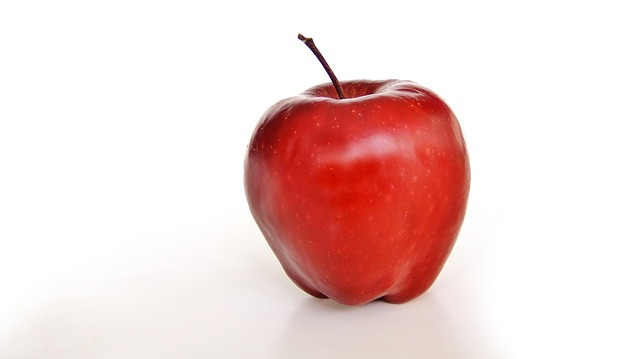µ GCSE | G.P.E. + K.E.

10 quick questions - for GCSE and iGCSE

10 minutes maximum! (can you do it in 5?)

(For all of these questions, the gravitational field strength of the Earth g is taken to be 10 N/kg)

1. What is the unit used to measure energy and work done?

• A. Watts (W)
• B. Newtons (N)
• C. Pascals (Pa)
• D. Joules (J)

2. Which of these is the correct formula for gravitational potential energy (G.P.E.)?

(g is the 'Gravitational Field Strength' of the Earth)

• A. G.P.E.= mass x g x height change
• B. G.P.E.= mass x height change
• C. G.P.E.= mass x height change ÷ g
• D. G.P.E.= g x height change ÷ mass

3. If I lift a school bag of mass 5kg onto a shelf 2m up, the increase in G.P.E. will be....

• A. 10 J
• B. 20 J
• C. 50 J
• D. 100 J

4. A 100 g apple falls from a tree. If it loses 2 J of G.P.E. What distance has it fallen?

• A. 0.002 m
• B. 2 m
• C. 5 m
• D. 500 cm5. To calculate kinetic energy (K.E.) we need the velocity (v) and the mass (m).

Using this notation, the formula for K.E. is:

 A. K.E. = ½ m v B. K.E. = m v C. K.E. = ½ m v 2 D. K.E. = m 2 v

Q6-8 This table shows the kinetic energy of different sports balls in a competition. What are the missing values?Type of Ball Mass Velocity Energy 6. Bowling ball 2 kg 3 m/s ? 3 J 6 J 9 J 18 J 7. Tennis ball 100 g 10 m/s ? A 5 J 10 J 1000 J 5000 J 8. Netball ? 0.1 kg 0.5 kg 1 kg 2 kg 2 m/s 1 J

9. A basketball of mass 0.6 kg is lifted to a height of 5 m. What is the G.P.E. gain at this height?

• A. 0.5 J
• B. 3 J
• C. 5 J
• D. 30 J

10. The ball is then dropped and all of this energy is eventually transferred (shifted) to kinetic energy.

Ignoring air friction, what would be the maximum velocity just before it hits the floor?

• A. 7.07 m/s
• B. 10 m/s
• C. 3.16 m/s
• D. 100 m/s